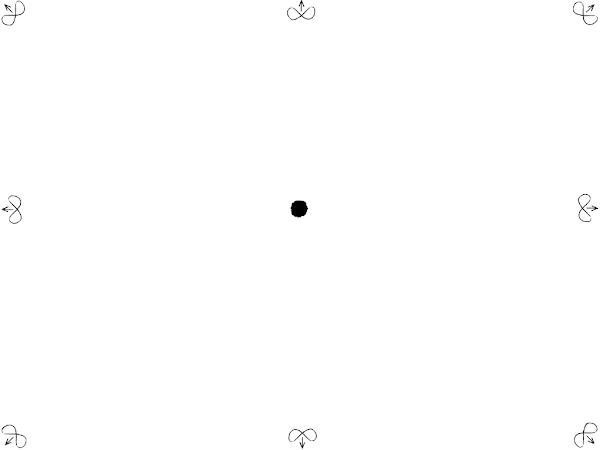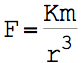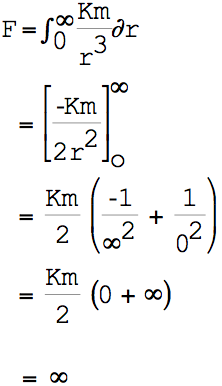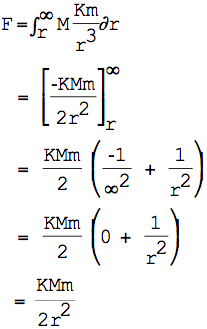## Wednesday, July 25, 2012

### TOLOS Chapter 3: Introduction to Gravity

Introduction to Gravity: Why stuff seems to be universally attracted to other stuff.
This chapter is part of a much bigger theory about where there is so much stuff and why all that stuff acts the way it does. It's just a theory. Writing things down and sharing it helps me think.

What you read here is the chapter as it existed on this date in 2012. For the live, always-updated version of this chapter, see here.

a universe, infinite and empty

Imagine a simple and traditional model of a universe as an infinite expanse of nothingness extending forever in 3 dimensions.

Here’s a 2-dimensional representation of that empty universe:

a universe, infinite and empty, except for one marble

Now imagine in this universe that there is a single bit of stuff, one very-small and very-solitary marble, surrounded infinitely in 3 dimensions by nothing. We don’t yet care how that marble got there; just imagine that it got there and it’s not going away any time soon.

Here’s our previous picture, with the marble added:but wait, an infinite universe cannot be empty; it is frothy

Now let’s go back to the pre-marble, infinite-3d-space representation of the universe. In that imagined universe space was empty, but that cannot be an accurate representation of space because it is constantly empty—there is nothing there. Recalling the primary postulates of Chapters 1 & 2 (the totalitarian and homogeneity principles), if there is nothing anywhere, then there is nothing anywhere to prevent anything from just showing up anywhere. In fact, the principles demand that, with nothing anywhere to contradict it, anything has an equal chance of being anywhere.

So let’s update the image of the empty universe to contain a snapshot of a random sampling of particles that have popped in (because nothing was there to stop them from popping in).

In the image (above), various sized particles occupy this space. The particles are represented by waves of varying length, with larger particles having a long wavelength and smaller particles having a shorter wavelength . But this is just an extremely momentary image of empty space, because all of the particles we see here are in contradiction with each other and with other particles that have just as much chance of showing up. This contradiction cannot stand, and so these particles cannot stand. They must go. They are extremely temporary.

And so it goes, ephemeral particles popping in and out, barely there at all. Empty space is never really empty, but is instead a very frothy and never-quite-empty space. 

a universe, infinite, empty, and frothy, except for one marble

Let’s take this new image of the empty universe, and put the marble back in.

This looks very much like we took the previous frothy image and just stuck a dot in the middle; but there are other changes: some of the ephemeral particles are no longer drawn. Because there is now a permanent bit of stuff there, its existence conflicts permanently with those now-missing ephemeral particles; those particles aren’t allowed, no matter how briefly, and so don’t show up here. (For simplicity, we are treating this marble as if it would perfectly conflict with, and so prevent, any particle that would occupy its space: an ideal mass. Most bits of stuff, even permanent stuff, don’t have such perfect interaction-with-everything and so would only have prevented some of these ephemeral particles, but not all.)

This animation should make it easier to find the ephemeral particles that are now missing:

effects of an infinite froth on one marble

In the previous image we can see a couple of sample ephemeral particles (a yellow one on the left, and a red one on the right) that are bumping up against our marble. Even though these two representative particles are extremely impermanent, they are still noticed. However briefly they may be considered to exist, they do have a momentum (represented by p) that is inversely proportional to length (aka “wavelength” or λ) of that ephemeral particle and some constant k1 according to the formula:

 p = k1 / λ

Consider a sample ephemeral particle of length λ interacting with our marble, and the space sphere (radius r) of all such ephemeral particles around the marble, as represented here:

Let’s define some values:

 m: This value represents the probability that this given marble object will interact with the ephemeral particles. A perfect value for m would interact with (and prevent overlap of) all ephemeral particles. We will begin to refer to this as the “mass” of the marble, and will explore many features of mass in later chapters. In this introduction, a mass of 1.0 would interact with all of the local ephemeral particles (where “interact” means either to be pushed by, or to prevent the existence of, depending on relative location), 0.0 would interact with none of them, and 0.5 would interact with half. k2: A constant representing how dense are these ephemeral particles in space; i.e., how many ephemeral particles exist in any point of space. (It will be many chapters and a lot of difficult math before we figure out the value of this constant.)

For any ephemeral particles terminating at that point of the sphere, we’d expect that ephemeral particle has an equally-likely chance of being aligned in any direction; and so the density of those that will also terminate on our marble at the center diminishes with the surface area of that sphere (4 π r^2).

What we want to know is the Force (F) of the ephemeral particle on our marble. Force is directly proportional to momentum, and so we’ll take a big shortcut and just treat the Force as a constant (k3) proportional to the momentum. 

Finally, the force of that particle on our marble is:(3.1)

Combining all of the constants (k1, k2, k3, 4, π) into a single constant K simplifies the formula to:(3.2)

Now consider the combined force in that one direction of all ephemeral particles as the distance from the marble goes from 0 to infinity:(3.3)

The result seems to be that for any real small marble in an infinite 3-dimensional universe, there is an infinite force pressing down on our marble from all directions, due to the 1/0^2 part of our equation. That’s an impossible amount of force! But consider that this is a very simplified approximation of a universe: we’re assuming that it can be representing by only 3 dimensions , and that the 3-dimensional space is identical in all directions and at all distances from the marble —these assumptions become increasingly invalid as we approach distance zero from the marble, and so our formula is less reliable as we get closer to distance zero, which is the cause of the infinity in our final calculation. The most we can say is that there seems to be a lot of pressure on a single item within an infinite empty space.

So it’s not clear what this infinity even means, or if it’s a meaningful value given the gross approximations made in this simple example. In any case it’s not a very useful situation because it’s not until there is at least one other marble in the universe that anything interesting happens. So let’s add another marble.

a universe, infinite, empty, and frothy, except for two marbles

Let’s now add a second marble.

As with the first marble, this second marble has prevented some of the ephemeral particles that were previously in our drawing. The following animation should help highlight the ephemeral particles which no longer have an opportunity to be:

Notice that most of these now-missing ephemeral particles never affected the first marble and so their absence will not be noticed . But there is one long red representative particle that used to impact the first marble and no longer does. It is particles like this, or rather the absence of particles like this, that we’ll explore in the next section.

Before proceeding, we must understand the severe limitations of the above representation of the two marbles and the space around them so that their poor representation does not distort our understanding. For one thing, in this drawing the two marbles are represented as large objects close together, when really they should be understood as point-sized marbles at a very great distance from one another. By drawing the particles as relatively close and large (with one larger than the other), it is easier to artistically represent some density of ephemeral particles that are affected by the marbles. In the math that follows, we’ll treat these as point-sized particles with different values of m and M instead of being different sizes .

effects of infinite froth on two marbles

This drawing shows the two marbles nearly point-sized along with a representative sample of the ephemeral particles that effect the marble on the left (m). Notice that the particles surround m uniformly from all sides and distances except for those longer particles that are blocked by the particle on the right (M). This means that there are more ephemeral particles pushing against m from the left than from the right. In the drawing a couple of particles are highlighted: the ones on the left that are not blocked by similar particles on the right. As we’ll see, it is those highlighted particles on the left that have the net effect on m due to M: they will act to push m toward M.

Let’s calculate the total force of those ephemeral particles that push from the left, but are not balanced by similar ephemeral particles pushing from the right.

Where the marbles are r distance apart, some of the ephemeral particles directly to the right of m with a wavelength longer than r are prevented from participating because of the existence of M, while to the left of m no particles of wavelength r or longer are prevented. So the net force on m is the total of all the particles to the left and with wavelength greater than r whose corresponding particle to the right would be prevented by M, where M is the probability that the right marble would be interacting with ephemeral particles.(3.4)

Let’s define the constant K/2 as a new constant G and rewrite our law of force as:(3.5)

interpreting the effects of infinite froth on two marbles as gravity

Our final formula probably looks familiar as Newton’s law of universal gravitation. Because of our derivation of the formula, we define it slightly differently:where:

 m: The mass of one of the objects, where “mass” represents the probability that this given object will interact with the ephemeral particles. M: The mass of the other object. r: The distance between the objects. G: The gravitational constant, a constant which is a rollup of other constants and geometric functions. F: The force pushing the masses together.

The difference between this formula and Newton’s is the interpretation. Newton interprets gravity as a fundamental force of attraction of objects toward each other, while we see gravity as a repulsive force resulting from “empty” space pushing from all directions, but pushing less strongly in the space between two objects. In both views the objects tend to get closer together, where in one view they are pulled together and in the other view they are pushed.

I.E. Even though we often think of gravity as something that attracts objects together, gravity is actually a repulsive force. Gravity is push, not pull.

brief consideration of two marbles in a confined geometry

Let’s briefly explore a differently-modeled universe, and see how a repulsive gravity would effect our two marbles there.

At the beginning of this chapter we assumed an infinite universe. Let’s now change that geometry such that the universe is not infinite but instead has edges. (Don’t ask how the universe may have edges, yet; we didn’t explain then why our assumed universe was infinite, so we won’t explain now why it is not.)

This is a drawing of a finite universe with two marbles, where those two marbles are relatively close to the edge of the universe. The drawing also shows a representative sample of ephemeral particles that are interacting with the marble on the left.

Notice that in this geometry there are a lot more ephemerals particles pushing against our marble from the right (i.e. from the center) than from the left (i.e. the edge).

If you were watching the two marbles in this universe, you would see them moving away from each other. If your complete knowledge of this universe was only what you saw of these two marbles, you would say that all of the objects are moving away from the center and this universe was expanding.

visual aid

This video (taken at the Exploratorium in San Francisco) is of a table containing a couple of slices of PVC tube being pushed around by a bunch of energized little balls that are propelled semi-randomly by the edges of the table.

In this video, the PVC slices represent our two marbles, the silver balls are our ephemeral particles, and the table is the finite universe. If you watch this video using a lot of imagination and pretend that the silver balls are invisible, so that you only see the PVC tubes, you might notice a couple of things:
1. The PVC tubes tend to get closer to each other over time, as if they are attracted to each other. This is a very jerky attraction, but smoothed out over time they do tend to come together like our two marbles experiencing gravity. 
2. The PVC tube pair tends to move toward the edge of the table, as if they are being pushed away from the center of our “expanding universe”.
conclusion

Starting with a few fundamental principles, assumptions about the configuration of a universe, and simplifying approximations, an inverse-squared mechanism is proposed that we call gravity. Gravity is a repulsive force. In some geometrical arrangements gravity appears to push together and make the overall configuration grow more compact; in other geometrical arrangements gravity is pushing objects apart and making the overall configuration expand.

questions to explore

Some of these questions will be examined in subsequent chapters. Some are still not understood.
• What is the value of G and the constituent constants of G. Is it enough to simply measure that value?
• Can the values for G and its constituent constants be derived from some fundamental principles?
• So we have a formula for gravity that looks like the formula we already have? So what? Does this different derivation and interpretation of the same old formula change anything for us? Does it provide any new predictions we can test?
• When we are no longer approximating at great distances, how does the formula change? How does the alteration of the nature of the space near a mass change the calculations for gravity?
• When 3 dimensions is no longer an accurate approximation (e.g. at small distances) how does the computation change?
• What about the other fundamental forces? Can they be derived in a similar way? Are they also repulsive?
• What is the nature of these ephemeral particles? Are they violating other laws, such as conservation of energy?
• How does this gravitational form of mass mesh with other forms, e.g. inertial, energy? Is there a related description of Newton’s First Law? Conservation of momentum? Conservation of angular momentum?
• Gravity is a scalar property that acts in a single direction. What about an aspect of matter interaction that uses more dimensions of space?
• Where did the space and dimensions come from in the first place?
• If 3 is just an approximation of dimensions, then what are dimensions and how many are there?
• Mechanical explanations for gravity, many with strong similarities to this theory, have been proposed for centuries, and have been all discredited (by all but a few eccentrics). Also, general relativity has explained the whole field. So why bother?
------------------------------------------------------------------------

Footnotes:

 The drawing shows more small particles than medium particles, more medium than large, and so on. I don’t have a good justification for drawing them that way except that at the time it felt right. It seemed that the larger a particle is, the likelier is the chance that somewhere in the space it occupies a smaller particle has also shown up to occupy that space--at whatever scale we look at it, the larger a particle is the smaller chance it has of showing up where some number of smaller particles have not already shown up. But the math performed later does not justify this assumption, although the longer particles do have a smaller momentum, and it is the momentum that is important to the calculations, so maybe a better drawing would show just as high a density of long particles as shorter ones, but they might appear more faint to represent the impact of each particle.

 These particles may be the same as the “virtual particles” commonly described in physics, and allowed by (and subject to) the uncertainty principle. I’m not sure. Maybe this is just a different way to look at it.

 Force is generally represented as momentum/time. In subsequent TOLOS chapters we’ll look into much further detail into what is meant by “time”. For the purposes of this calculation just imagine that the time interval is some fixed value: if we choose a longer value then that time divisor is larger, but there will also be proportionally more virtual particles, so the ratio of force to momentum won’t change, and F∝p will suffice.

 In other chapters we’ll discuss how our primary postulates limit us from accepting that there’s something special about 3 dimensions (why not 4, or 12, or 2471?) except that at relatively large scales and a scalar force like we’re discussing here, 3 dimensions is the minimum for something interesting to happen and is a valid approximations for higher-dimensions.

 The drawing around the single marble is inaccurate in a lot of ways, only one of which is how it portrays the space around the marble. Consider that the drawing does show that some of the ephemeral particals are missing near the marble. A better portrayal would represent the space near the particle being different than the space far away, because the marble has effected by removing the possibilities of what might ephemerally exist there. This might better be conveyed by showing a lightening of the gray (where gray was meant to represent “lots of ephemeral stuff going on here”), or perhaps a stretching of the area around the marble to represent that the stuff that is a thick froth elsewhere has been stretched thinner near the marble. As the drawing become less accurate close to the one bit of permanent stuff, so does the formula become less accurate in this region.

 It’s a simplification to say that the missing particles at a distance have no effect at all on the first marble. They do change the nature of the space around that new marble, which will have cascading effects on the first particle. But for the approximations here we’re assuming a large relative distance between the two marbles and so we accept that the effect of the area immediately around the new marble has relatively little effect on our overall calculations.

 In a following chapter or exercise, we will show that representing different values of m and M as either single points, or as different size spheres with those single points as their center, are equivalent when calculating the effect of each marble on the other at a great distance. To see this, it may help to picture a real-sized marble as a collection of many point-sized sub-marbles spread around within the marble-sized sphere, where each point-sized sub-marble is itself at a relatively great distance from the others.

 This table was probably intended to demonstrate Brownian motion, which is jerky. To redesign this table better for our purposes, the silver balls would need to be much much smaller related to the PVC slices, which should result in a much smoother movement.

## Tuesday, July 17, 2012

### The open source process works… eventually

I find this story fascinating, but I suspect that nobody else will.

About a year and a half ago I filed this bug with the open-source chromium project, which is the code behind the Google Chrome browser. (The bug, in case you care, was that this mp3 file was sounding like crap [listen to crap] when played in Chrome on my Mac.)

Here's the long story of this bug fix:
• 2011-01-14 – I enter bug
• 2011-03-11 – a chromium developer comments that it’s a duplicate of another bug (btw, turns out not to be)
• 2011-12-16 – another chromium developer cannot reproduce the problem
• 2011-12-18 – I further clarify how to demonstrate the problem
• 2011-12-19 – chromium dev confirms they can reproduce the problem, and confirms my original hint that it’s probably an issue with ffmpeg [a different open source project that is built into chromium]
• 2011-12-19 – a google engineer has a look
• 2012-01-04 – the bug is passed from chromium to the ffmpeg open source project
• 2012-05-16 – the bug is fixed in ffmpeg
• 2012-05-21 – the ffmpeg bug fix is pulled into chromium
• 2012-07-?? – sometime in this period my Chrome updates itself with a new version that fixes the bug
Here's the short story:
• January 2011 – I find and report a bug in my browser
• July 2012 – the bug is no longer in my browser
So the free and open source process worked. Actually at least two (maybe three) open source projects had to come together to make this happen. I entered a bug, did nothing else (and certainly paid nothing), and the fix just happened. I find that amazing.

On the other hand, it took 18 months for the bug (admittedly not the most important bug in the world) to get fixed. 18 months to fix a bug. I find that amazing, too.

… if you liked that story (you pitiful soul), you’ll really love this poem about open source.

## Friday, July 13, 2012

### The Most Honest Driver in The World

You can call this driver a lot of things, but not a hypocrite.

Shame on me, once again, for using a car, for lacking this driver's honesty, and for taking a picture while I'm driving.